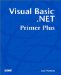# Other Array Properties and Methods

### Other Array Properties and Methods

A number of other properties and methods are available for you to use with arrays. A partial list of some of the more useful array properties and methods are presented in the following sections.

#### Length

The syntax for this array property is

`  ElementCount  = MyArray.Length `

The property is the total number of elements in the array. Note that this is not the same as the upper bound index of an array. For example, if you had an array defined as

` Dim MyArray(10, 10) As Integer `

ElementCount would equal 121 for MyArray() . That's because each dimension for the array runs from 0 through 10, or 11 elements.

#### Rank

The syntax for this property is

`  NumDimensions  = MyArray.Rank `

The property is the number of dimensions for the array. The comment tells the Rank for each array.

` Dim MyArray(10,10) As Integer    ' Rank = 2  Dim YourArray(11) As Integer     ' Rank = 1 `

In some circumstances, knowing the Rank in conjunction with symmetrical arrays can be useful in loop processing.

#### Clear

The syntax for this method is

` Array.Clear(  ArrayToClear, StartAtIndex, NumberToClear  ) `

The first parameter is the name of the array you want to clear. The second parameter is the starting index to be cleared. Normally, this would be element 0, but this and the third parameter enable you to partially clear an array. The third parameter is the number of elements that you want to clear. For example,

` Dim X(100) As Integer  Array.Clear(X, 0, 10) `

would clear out elements 0 through 9. Please note, however, that the statement

` Array.Clear(X, 3, 3) `

clears out elements X(3) , X(4) , and X(5) . Therefore, keep in mind that the middle parameter is an index number, not element position.

#### Copy

This method makes two forms available to you. The first version is

` Array.Copy(  SourceArray, DestinationArray, NumberToCopy  ) `

This version says to copy NumberToCopy elements from the SourceArray into the DestinationArray . Note that the two arrays must have the same Rank .

The second version is

` Array.Copy(  SourceArray, SourceStart, DestinationArray,  _  DestinationStart, NumberToCopy  ) `

In this case, you can copy a certain number of elements, starting at a position other than zero. You also can place the source elements in the destination array at a different location. For example,

` Array.Copy(X, 10, Y, 20, 50) `

says to copy 50 elements from the X() array starting at index 10 and place them in the Y() array starting at index 20.

#### GetLength

The syntax for GetLength is

`  NumberOfElements  = MyArray.GetLength(  WhichDimension  ) `

This method yields the number of elements in the array for the dimension specified, as stored in NumberOfElements . For example,

` Dim NumEl, X(10), Y(10,20) As Integer  NumEl = X.GetLength(0) NumEl = Y.GetLength(1) `

The first statement would find NumEl equaling 11, whereas the second call would find NumEl equal to 21.

This method could be useful with the Rank property of an array. For example, if an array is passed in and you're unsure of its size, Rank could tell you how many dimensions it has and GetLength could tell you the size of each dimension.

#### Reverse

This method has two flavors. The first is

` Array.Reverse(  ArrayToReverse  ) `

This method flips the array so that the last value in the array becomes the first value in the array. In other words, if your array exists in ascending sorted order, after calling this method, the array will be sorted in descending order.

The second version has the syntax:

` Array.Reverse(  ArrayToReverse, StartIndex, Length  ) `

In this version, you can reverse just part of the array starting with the StartIndex index of the array, reversing Length elements. For example,

` Dim X(10) As Integer Array.Reverse(X, 3, 3) `

would flip positions for elements X(3) , X(4) , and X(5) .

Other array methods and properties are available to you. I've presented some of the more common ones in this section. You can use the Visual Basic .NET online help to explore the others.

All of these array-processing features are made possible because Visual Basic .NET creates arrays as objects of the Array class. This makes array processing different from other dialects of Basic you may have used. However, it also makes using arrays in Visual Basic .NET very easy.Visual Basic .NET Primer Plus
ISBN: 0672324857
EAN: 2147483647
Year: 2003
Pages: 238
Authors: Jack Purdum

Similar book on Amazon